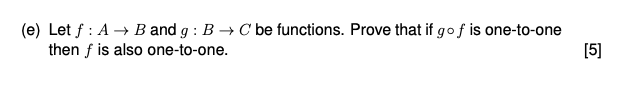# Question Solved1 Answer(e) Let f : A + B and g: B C be functions. Prove that if gof is one-to-one then f is also one-to-one.Transcribed Image Text: (e) Let f : A + B and g: B C be functions. Prove that if gof is one-to-one then f is also one-to-one. 
More
Transcribed Image Text: (e) Let f : A + B and g: B C be functions. Prove that if gof is one-to-one then f is also one-to-one.